# Ulps Plots Reveal Math Function Accuracy

"ULP" stands for "unit in the last place." An ulps plot samples a fundamental math function such as $\sin{x}$, or a more esoteric function like a Bessel function. The samples are compared with more accurate values obtained from a higher precision computation. A plot of the accuracy, measured in ulps, reveals valuable information about the underlying algorithms.

### Contents

#### fdlibm

libm is the library of elementary math functions that supports the C compiler. fdlibm is "freely distributable" source for libm developed and put into the public domain over 25 years ago by K. C. Ng and perhaps a few others at Sun Microsystems. I wrote about fdlibm in our newsletter in 2002, The Tetragamma Function and Numerical Craftsmanship.

Mathematically fdlibm shows immaculate craftsmanship. We still use it today for our elementary transcendental functions. And I suspect all other mathematical software projects do as well. If they don't, they should.

#### ulps plots

ulps(x) is the distance from x to the next larger floating point number. It's the same as eps(x).

To assess the accuracy of a computed value

y = f(x)


compare it with the more accurate value obtained from the Symbolic Math Toolbox

Y = f(sym(x,'f'))


The 'f' flag says to convert x to a sym exactly, without trying to guess that it is an inverse power of 10 or the sqrt of something. The relative error in y, measured in units in the last place, is then

u = (y - Y)/eps(abs(Y))


Since this is relative error, it is a stringent measure near the zeros of f(x).

If y is the floating point number obtained by correctly rounding Y to double precision, then

-0.5 <= u <= 0.5


This is the best that can be hoped for. Compute the exact mathematical value of f(x) and make a single rounding error to obtain the final result. Half-ulp accuracy is difficult to obtain algorithmically, and too expensive in execution time. All of the functions in MATLAB that are derived from fdlibm have better than one-ulp accuracy.

-1.0 < u < 1.0


Each of the following plots involves 100,000 random arguments x, uniformly distributed in an appropriate interval.

#### sin

We see about 0.8 ulp accuracy from this sample. That's typical.

Argument reduction is the first step in computing $\sin{x}$. An integer multiple $n$ of $\pi/2$ is subtracted from the argument to bring it into the interval

$$-\frac{\pi}{4} \le x - n \frac{\pi}{2} \le \frac{\pi}{4}$$

Then, depending upon whether $n$ is odd or even, a polynomial approximation of degree 13 to either $\sin$ or $\cos$ gives the nearly correctly rounded result for the reduced argument, and hence for the original argument. The ulps plot shows a slight degradation in accuracy at odd multiples of $\pi/4$, which are the extreme points for the polynomial approximations.

It is important to note that the accuracy is better than one ulp even near the end-points of the sample interval, $0$ and $2\pi$. This is where $\sin{x}$ approaches $0$ and the approximation must follow carefully so that the relative error remains bounded.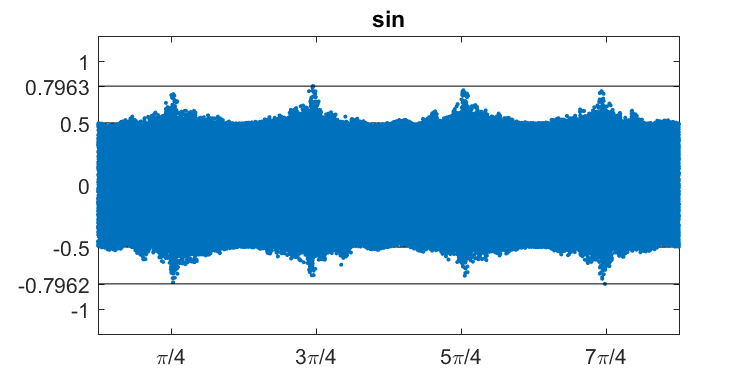#### tan

Again, roughly 0.8 ulp accuracy.

Similar argument reduction results in similar behavior near odd multiples of $\pi/4$. In between these points, at $\pi/2$ and $3\pi/2$, $\tan{x}$ has a pole and the approximation must follow suit. The algorithm uses a reciprocal and the identity

$$\tan x = -1/\tan{(x+\frac{\pi}{2})}$$

This comes close to dividing by zero as you approach a pole, but the resulting approximation remains better than one ulp.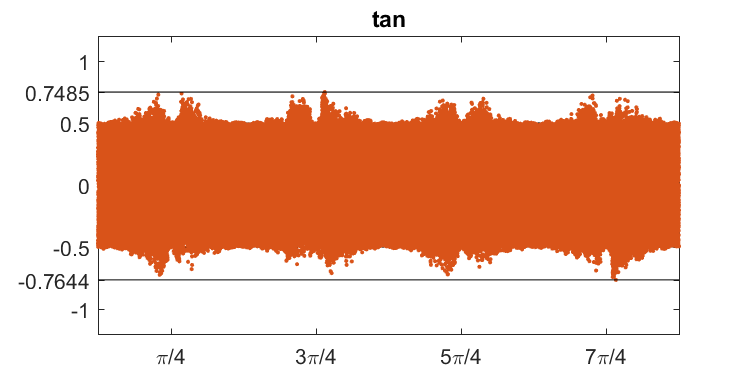#### atan

Good to within slightly more than 0.8 ulp. The underlying approximation is a piecewise polynomial with breakpoints at a few multiples of 1/16 that are evident in the plot and marked on the axis.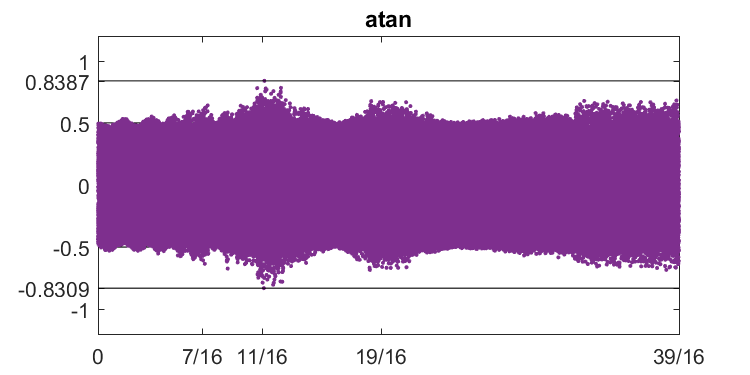#### exp

About the same accuracy as the previous plots.

The argument reduction involves the key value

$$r = \ln{2} \approx 0.6931$$

and the identity

$$\exp{x} = 2^n \exp{(x-n r)}$$

The resulting ulps plot shows the extremes of the error at odd multiples of $r/2$.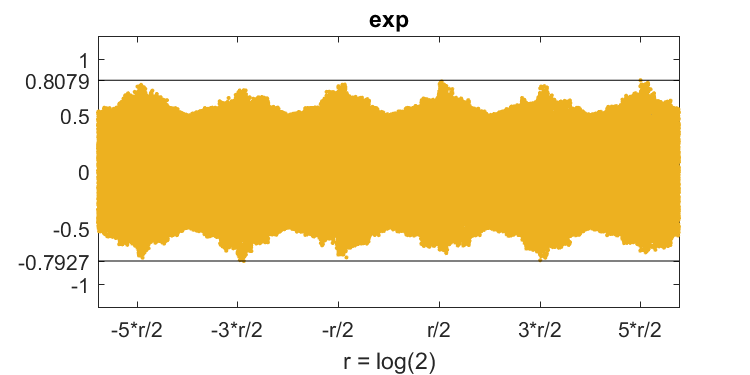#### Lambert W

Now for two functions that are not in fdlibm. If you follow this blog, you might have noticed that I am a big fan of the Lambert W function. I blogged about it a couple of years ago, The Lambert W Function. The Wikipedia article is excellent, Lambert W Function. And, you cn just Google "lambert w function" for many more interesting links.

The Lambert W function is not available in MATLAB itself. The Symbolic Math Toolbox has an @double method that accesses the symbolic code for type double arguments. Or, my blog post mentioned above has some simple (but elegant, if I do say so myself) code.

Neither code is one-ulp accurate. The primary branch of the function has a zero at the origin. As we get near that zero, the relative error measured in ulps is unbounded. The absolute accuracy is OK, but the relative accuracy is not. In fact, you might see a billion ulps error. That's a gigaulp, or gulp for short.

As lambertw(x) crosses a power of two, the unit in the last place, eps(lambertw(x)), jumps by a factor of two. Three of these points are marked by ticks on the x-axis in the ulps plot.

   for a = [1/8 1/4 1/2]
z = fzero(@(x) lambertw(x)-a,.5)
end

z =
0.1416
z =
0.3210
z =
0.8244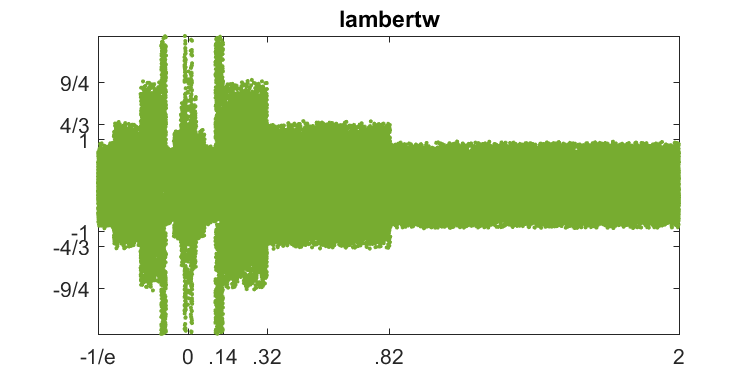#### Bessel functions

Our codes for Bessel functions have fairly good, although not one-ulp, absolute accuracy. But they do not have high relative accuracy near the zeros. Here are the first five zeros, and an ulps plot covering the first two, for $J_0(x)$, the zero-th order Bessel function of the first kind.

   for a = (1:5)*pi
z = fzero(@(x) besselj(0,x), a)
end

z =
2.4048
z =
5.5201
z =
8.6537
z =
11.7915
z =
14.9309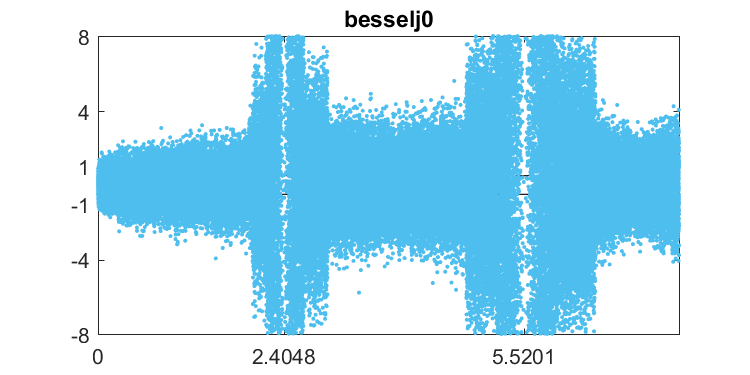#### erfinv

Here is the inverse of the error function. It looks very interesting, but I haven't investigated it yet.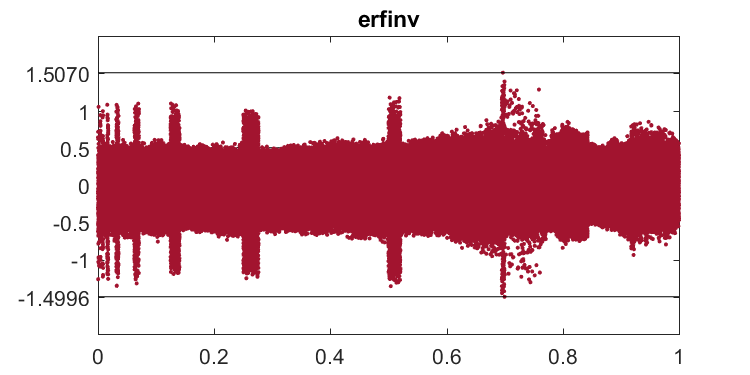#### Code

I have recently updated Cleve's Laboratory in the MATLAB Central file exchange. The latest version includes ulpsapp.m, which generates the ulps plots in this blog.

Published with MATLAB® R2017a

|